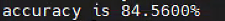# Different result between Linux and Window

I change a particular parameter in resent-18 and use it to infer the test set. And I got two extremely different result. I guess the reason is the difference of representation between Linux and Windows, but I’m not sure why is that. My question is what could I do to make these two results the same. The result in Linux is hereand Windows is hereThe code in these two operating systems is almost the same (e.g. batch_size) except for `num_work` in `DataLoader`. My code is below

``````import torch
from torchvision import datasets, transforms
import time
device = torch.device('cuda:0' if torch.cuda.is_available() else 'cpu')

# in order to modify specific parameter
flag = 0
for layerPara in model.parameters():
if flag < 3:
flag = flag + 1
continue
layerPara = -3e+37
break

model.to(device)
model.eval()
accuracy = 0.0
correctNum = 0
data_transforms = transforms.Compose([
transforms.ToTensor(),
transforms.Normalize((0.5, 0.5, 0.5), (0.5, 0.5, 0.5))
])
image_datasets = datasets.CIFAR10(root='./data', train=False,
shuffle=True, num_workers=0, prefetch_factor=2)
since = time.time()
data = data.to(device)
target = target.to(device)
output = model(data)
pred = output.data.max(1, keepdim=True)  # get the index of the max log-probability
correctNum += pred.eq(target.data.view_as(pred)).cpu().sum()  # 对预测正确的数据个数进行累加
print("accuracy is {:.4f}%".format(accuracy * 100))
time_elapsed = time.time() - since
``````

An accuracy of 13% suggests that something is either not initialized or there is a preprocessing mismatch between the models. Can you check both versions on a single input image and observe the outputs (even at intermediate parts of the model if necessary) to see where they differ?

Thank you for your reply. According to your idea, I change batch_size = 1 to calculate a single output. I got two different outputs in these two OS. Linux is this

and Windows is this

Strangely, when I change the particular parameter in the code I post to 3e+37, both Linux and Windows have the same result.

You might want to check if the model parameters are loaded correctly in Windows. As a sanity check you can also try printing a few values of the input before it is passed to the model in both cases.

After the parameter is modified, I print the value and verify it. This problem is too confusing.